Chapter 7. Stoichiometry of Chemical Reactions

# 7.1 Reaction Stoichiometry

### Learning Objectives

By the end of this section, you will be able to:

• Explain the concept of stoichiometry as it pertains to chemical reactions
• Use balanced chemical equations to derive stoichiometric factors relating amounts of reactants and products
• Perform stoichiometric calculations involving mass, moles, and solution molarity

A balanced chemical equation provides a great deal of information in a very succinct format. Chemical formulas provide the identities of the reactants and products involved in the chemical change, allowing classification of the reaction. Coefficients provide the relative numbers of these chemical species, allowing a quantitative assessment of the relationships between the amounts of substances consumed and produced by the reaction. These quantitative relationships are known as the reaction’s stoichiometry, a term derived from the Greek words stoicheion (meaning “element”) and metron (meaning “measure”). In this module, the use of balanced chemical equations for various stoichiometric applications is explored.

The general approach to using stoichiometric relationships is similar in concept to the way people go about many common activities. Food preparation, for example, offers an appropriate comparison. A recipe for making eight pancakes calls for 1 cup pancake mix, $\frac{3}{4}$ cup milk, and one egg. The “equation” representing the preparation of pancakes per this recipe is

$1 \;\text{cup mix} + \frac{3}{4} \;\text{cup milk} + 1 \;\text{egg} \longrightarrow 8 \;\text{pancakes}$

If two dozen pancakes are needed for a big family breakfast, the ingredient amounts must be increased proportionally according to the amounts given in the recipe. For example, the number of eggs required to make 24 pancakes is

$24 \;\rule[0.5ex]{4em}{0.1ex}\hspace{-4em}\text{pancakes} \times \frac{1 \;\text{egg}}{8 \;\rule[0.25ex]{3em}{0.1ex}\hspace{-3em}\text{pancakes}} = 3 \;\text{eggs}$

Balanced chemical equations are used in much the same fashion to determine the amount of one reactant required to react with a given amount of another reactant, or to yield a given amount of product, and so forth. The coefficients in the balanced equation are used to derive stoichiometric factors that permit computation of the desired quantity. To illustrate this idea, consider the production of ammonia by reaction of hydrogen and nitrogen:

$\text{N}_2(g) + 3\text{H}_2(g) \longrightarrow 2\text{NH}_3(g)$

This equation shows ammonia molecules are produced from hydrogen molecules in a 2:3 ratio, and stoichiometric factors may be derived using any amount (number) unit:

$\frac{2 \;\text{NH}_3 \;\text{molecules}}{3 \;\text{H}_2 \;\text{molecules}} \;\text{or} \;\frac{2 \;\text{doz NH}_3 \;\text{molecules}}{3 \;\text{doz H}_2 \;\text{molecules}} \;\text{or} \;\frac{2 \;\text{mol NH}_3 \;\text{molecules}}{3 \;\text{mol H}_2 \;\text{molecules}}$

These stoichiometric factors can be used to compute the number of ammonia molecules produced from a given number of hydrogen molecules, or the number of hydrogen molecules required to produce a given number of ammonia molecules. Similar factors may be derived for any pair of substances in any chemical equation.

### Example 1

How many moles of I2 are required to react with 0.429 mol of Al according to the following equation (see Figure 1)?

$2\text{Al} + 3\text{I}_2 \longrightarrow 2\text{AlI}_3$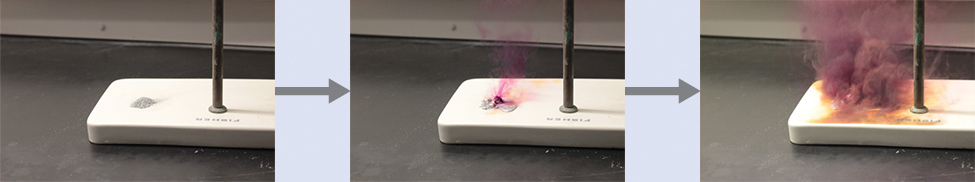Figure 1. Aluminum and iodine react to produce aluminum iodide. The heat of the reaction vaporizes some of the solid iodine as a purple vapor. (credit: modification of work by Mark Ott)

Solution
Referring to the balanced chemical equation, the stoichiometric factor relating the two substances of interest is $\frac{3 \;\text{mol I}_2}{2 \;\text{mol Al}}$. The molar amount of iodine is derived by multiplying the provided molar amount of aluminum by this factor: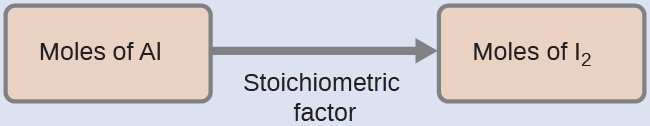$\begin{array}{r @{{}={}} l} \text{mol I}_2 & 0.429 \;\rule[0.5ex]{3.25em}{0.1ex}\hspace{-3.25em}\text{mol Al} \times \frac{3 \;\text{mol I}_2}{2 \;\rule[0.25ex]{2em}{0.1ex}\hspace{-2em}\text{mol Al}} \\[1em] & 0.644 \;\text{mol I}_2 \end{array}$

Test Yourself
How many moles of Ca(OH)2 are required to react with 1.36 mol of H3PO4 to produce Ca3(PO4)2 according to the equation $3\text{Ca(OH)}_2 + 2\text{H}_3 \text{PO}_4 \longrightarrow \text{Ca}_3 \text{(PO}_4)_2 + 6\text{H}_2 \text{O}$?

2.04 mol

### Example 2

How many carbon dioxide molecules are produced when 0.75 mol of propane is combusted according to this equation?

$\text{C}_3 \text{H}_8 + 5\text{O}_2 \longrightarrow 3\text{CO}_2 + 4\text{H}_2 \text{O}$

Solution
The approach here is the same as for Example 1, though the absolute number of molecules is requested, not the number of moles of molecules. This will simply require use of the moles-to-numbers conversion factor, Avogadro’s number.

The balanced equation shows that carbon dioxide is produced from propane in a 3:1 ratio:

$\frac{3 \;\text{mol CO}_2}{1 \;\text{mol C}_3 \text{H}_8}$

Using this stoichiometric factor, the provided molar amount of propane, and Avogadro’s number,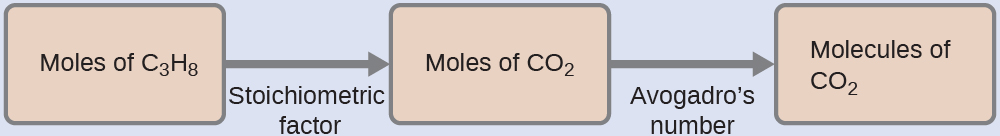$0.75 \;\rule[0.5ex]{4.5em}{0.1ex}\hspace{-4.5em}\text{mol C}_3 \text{H}_8 \times \frac{3 \;\rule[0.25ex]{3em}{0.1ex}\hspace{-3em} \text{mol CO}_2}{1 \;\rule[0.5ex]{3em}{0.1ex}\hspace{-3em}\text{mol C}_3 \text{H}_8} \times \frac{6.022 \times 10^{23} \;\text{CO}_2 \;\text{molecules}}{\rule[0.25ex]{3em}{0.1ex}\hspace{-3em} \text{mol CO}_2} = 1.4 \times 10^{24} \text{CO}_2 \;\text{molecules}$

Test Yourself
How many NH3 molecules are produced by the reaction of 4.0 mol of Ca(OH)2 according to the following equation:

$(\text{NH}_4)_2 \text{SO}_4 + \text{Ca(OH)}_2 \longrightarrow 2\text{NH}_3 + \text{CaSO}_4 + 2\text{H}_2 \text{O}$
4.8 × 1024 NH3 molecules

These examples illustrate the ease with which the amounts of substances involved in a chemical reaction of known stoichiometry may be related. Directly measuring numbers of atoms and molecules is, however, not an easy task, and the practical application of stoichiometry requires that we use the more readily measured property of mass.

### Example 3

What mass of sodium hydroxide, NaOH, would be required to produce 16 g of the antacid milk of magnesia [magnesium hydroxide, Mg(OH)2] by the following reaction?

$\text{MgCl}_2(aq) + 2\text{NaOH}(aq) \longrightarrow \text{Mg(OH)}_2(s) + \text{NaCl}(aq)$

Solution
The approach used previously in Example 1 and Example 2 is likewise used here; that is, we must derive an appropriate stoichiometric factor from the balanced chemical equation and use it to relate the amounts of the two substances of interest. In this case, however, masses (not molar amounts) are provided and requested, so additional steps of the sort learned in the previous chapter are required. The calculations required are outlined in this flowchart: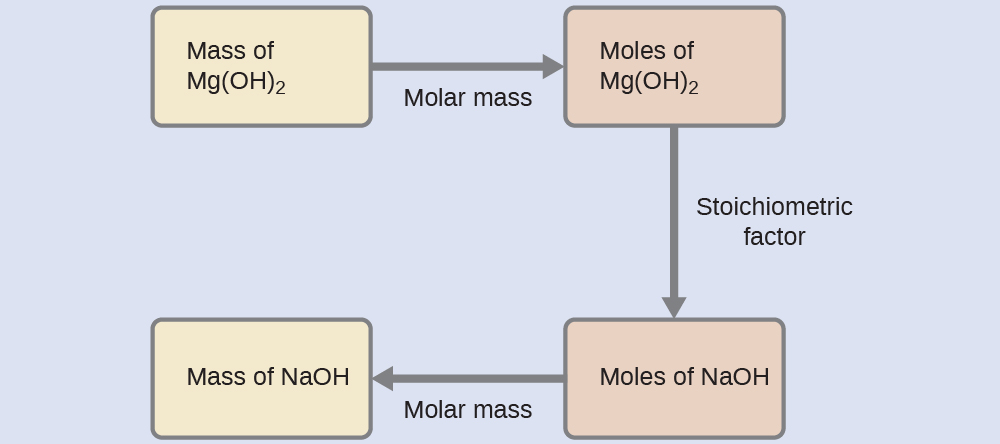$16 \;\rule[0.5ex]{5em}{0.1ex}\hspace{-5em}\text{g Mg(OH)}_2 \times \frac{1 \;\rule[0.25ex]{4.75em}{0.1ex}\hspace{-4.75em}\text{mol Mg(OH)}_2}{58.3197 \;\rule[0.25ex]{3.5em}{0.1ex}\hspace{-3.5em}\text{g Mg(OH)}_2} \times \frac{2 \;\rule[0.25ex]{3.5em}{0.1ex}\hspace{-3.5em}\text{mol NaOH}}{1 \;\rule[0.25ex]{4.5em}{0.1ex}\hspace{-4.5em}\text{mol Mg(OH)}_2} \times \frac{39.9971 \;\text{g NaOH}}{1 \;\rule[0.25ex]{3.25em}{0.1ex}\hspace{-3.25em}\text{mol NaOH}} = 22 \;\text{g NaOH}$

Test Yourself
What mass of gallium oxide, Ga2O3, can be prepared from 29.0 g of gallium metal? The equation for the reaction is $4 \text{Ga} + 3\text{O}_2 \longrightarrow 2\text{Ga}_2 \text{O}_3.$

39.0 g

### Example 4

What mass of oxygen gas, O2, from the air is consumed in the combustion of 702 g of octane, C8H18, one of the principal components of gasoline?

$2\text{C}_8 \text{H}_{18} + 25\text{O}_2 \longrightarrow 16\text{CO}_2 + 18\text{H}_2 \text{O}$

Solution
The approach required here is the same as for the Example 3, differing only in that the provided and requested masses are both for reactant species.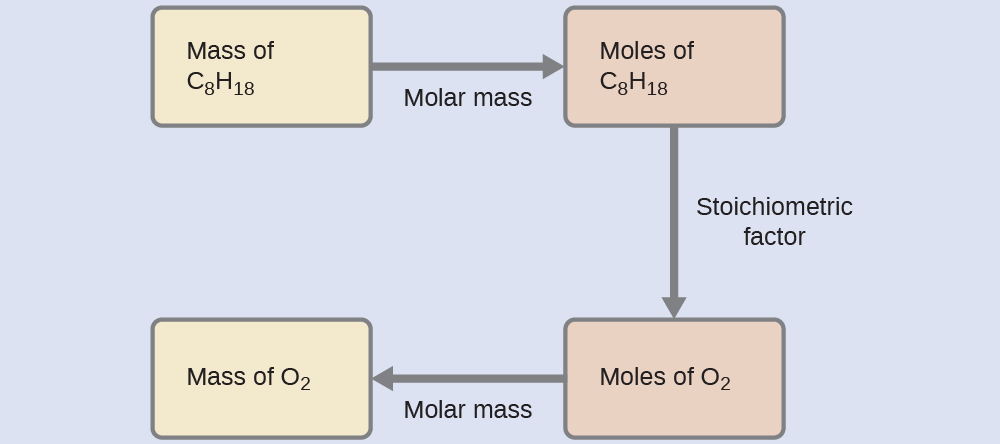$702 \;\rule[0.5ex]{3.5em}{0.1ex}\hspace{-3.5em}\text{g C}_8 \text{H}_{18} \times \frac{1 \;\rule[0.25ex]{3.5em}{0.1ex}\hspace{-3.5em}\text{mol C}_8 \text{H}_{18}}{114.231 \;\rule[0.25ex]{2.75em}{0.1ex}\hspace{-2.75em}\text{g C}_8 \text{H}_{18}} \times \frac{25 \;\rule[0.25ex]{2.5em}{0.1ex}\hspace{-2.5em}\text{mol O}_2}{2 \;\rule[0.25ex]{3.5em}{0.1ex}\hspace{-3.5em}\text{mol C}_8 \text{H}_{18}} \times \frac{31.9988 \;\text{g O}_2}{\rule[0.25ex]{2.5em}{0.1ex}\hspace{-2.5em}\text{mol O}_2} = 2.46 \times 10^3 \;\text{g O}_2$

Test Yourself
What mass of CO is required to react with 25.13 g of Fe2O3 according to the equation

$\text{Fe}_2 \text{O}_3 + 3\text{CO} \longrightarrow 2\text{Fe} + 3\text{CO}_2$

13.22 g

These examples illustrate just a few instances of reaction stoichiometry calculations. Numerous variations on the beginning and ending computational steps are possible depending upon what particular quantities are provided and sought (volumes, solution concentrations, and so forth). Regardless of the details, all these calculations share a common essential component: the use of stoichiometric factors derived from balanced chemical equations. Figure 2 provides a general outline of the various computational steps associated with many reaction stoichiometry calculations.

### Airbags

Airbags (Figure 3) are a safety feature provided in most automobiles since the 1990s. The effective operation of an airbag requires that it be rapidly inflated with an appropriate amount (volume) of gas when the vehicle is involved in a collision. This requirement is satisfied in many automotive airbag systems through use of explosive chemical reactions, one common choice being the decomposition of sodium azide, NaN3. When sensors in the vehicle detect a collision, an electrical current is passed through a carefully measured amount of NaN3 to initiate its decomposition:

$2 \text{NaN}_3(s) \longrightarrow 3\text{N}_2(g) + 2\text{Na}(s)$

## More Worked Out Problems

### Example 5

How many molecules of SO3 are needed to react with 144 molecules of Fe2O3 given this balanced chemical equation?

Fe2O3(s) + 3SO3(g) $\longrightarrow$ Fe2(SO4)3

Solution

We use the balanced chemical equation to construct a conversion factor between Fe2O3 and SO3. The number of molecules of Fe2O3 goes on the bottom of our conversion factor so it cancels with our given amount, and the molecules of SO3 go on the top. Thus, the appropriate conversion factor is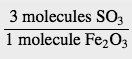Starting with our given amount and applying the conversion factor, the result is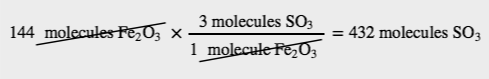We need 432 molecules of SO3 to react with 144 molecules of Fe2O3.

Test Yourself

How many molecules of H2 are needed to react with 29 molecules of N2 to make ammonia if the balanced chemical equation is N2 + 3H2 $\longrightarrow$ 2NH3?

87 molecules

### Example 6

How many molecules of NH3 can you make if you have 228 atoms of H2?

Solution

From the formula, we know that one molecule of NH3 has three H atoms. Use that fact as a conversion factor: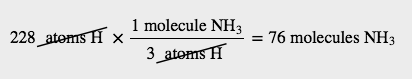Test Yourself

How many molecules of Fe2(SO4)3 can you make from 777 atoms of S?

259 molecules

### Example 7

How many moles of HCl will be produced when 249 g of AlCl3 are reacted according to this chemical equation?  The molar mass of AlCl3 is 133.34 g/mol.

2 AlCl3 + 3 H2O(ℓ) $\longrightarrow$ Al2O3 + 6 HCl(g)

Solution

We will do this in two steps: convert the mass of AlCl3 to moles and then use the balanced chemical equation to find the number of moles of HCl formed. The molar mass of AlCl3 is 133.34 g/mol, which we have to invert to get the appropriate conversion factor:

$249 \;\rule[0.5ex]{0.5em}{0.1ex}\hspace{-0.5em}\text{g AlCl}_3 \times \frac{1 \;\text{mol AlCl}_3}{133.34\;\rule[0.5ex]{0.5em}{0.1ex}\hspace{-0.5em}\text{g AlCl}_3} = \underline{1.86}74 \;\text{mol of aluminium chloride with 3 sig figs}$

Now we can use this quantity to determine the number of moles of HCl that will form. From the balanced chemical equation, we construct a conversion factor between the number of moles of AlCl3 and the number of moles of HCl:

$\frac{6 \; \text{mol HCl}}{2 \; \text{mol AlCl}_3}$

Applying this conversion factor to the quantity of AlCl3, we get

$\underline{1.86}74 \;\rule[0.5ex]{1.25em}{0.1ex}\hspace{-1.25em}\text{mol AlCl}_3 \times \frac{6 \;\text{mol HCl}}{2\;\rule[0.5ex]{1.25em}{0.1ex}\hspace{-1.25em}\text{mol AlCl}_3} =5.60 \;\text{mol HCl}$

Alternatively, we could have done this in one line:

$249 \;\rule[0.5ex]{0.5em}{0.1ex}\hspace{-0.5em}\text{g AlCl}_3 \times \frac{1 \;\rule[0.5ex]{1.25em}{0.1ex}\hspace{-1.25em}\text{mol AlCl}_3}{133.34\;\rule[0.5ex]{0.5em}{0.1ex}\hspace{-0.5em}\text{g AlCl}_3} \times \frac{6 \;\text{mol HCl}}{2\;\rule[0.5ex]{1.25em}{0.1ex}\hspace{-1.25em}\text{mol AlCl}_3}=5.60 \;\text{mol HCl}$

The last digit in our final answer is slightly different because of rounding differences, but the answer is essentially the same.

Test Yourself

How many moles of Al2O3 will be produced when 23.9 g of H2O are reacted according to this chemical equation?

2 AlCl3 + 3 H2O(ℓ) $\longrightarrow$ Al2O3 + 6 HCl(g)

0.442 mol

### Example 8

How many grams of NH3 will be produced when 33.9 mol of H2 are reacted according to this chemical equation?  Use 17.03 g/mol as the molar mass of NH3.

N2(g) + 3 H2(g) $\longrightarrow$ 2 NH3(g)

Solution

The conversions are the same, but they are applied in a different order. Start by using the balanced chemical equation to convert to moles of another substance and then use its molar mass to determine the mass of the final substance. In two steps, we have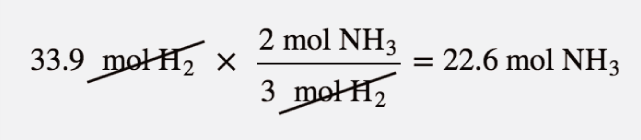Now, using the molar mass of NH3, which is 17.03 g/mol, we get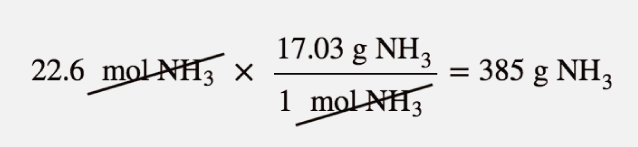Test Yourself

How many grams of N2 are needed to produce 2.17 mol of NH3 when reacted according to this chemical equation?

N2(g) + 3 H2(g) $\longrightarrow$ 2 NH3(g)

30.4 g (Note: here we go from a product to a reactant, showing that mole-mass problems can begin and end with any substance in the chemical equation.)

### Example 9

What mass of Mg will be produced when 86.4 g of K are reacted?  Use 39.09 g/mol as the molar mass of potassium and 24.31 g/mol as the molar mass of magnesium.

MgCl2(s) + 2 K(s) $\longrightarrow$ Mg(s) + 2 KCl(s)

Solution

We will simply follow the steps

mass K $\longrightarrow$ mol K $\longrightarrow$ mol Mg $\longrightarrow$ mass Mg

In addition to the balanced chemical equation, we need the molar masses of K (39.09 g/mol) and Mg (24.31 g/mol). In one line,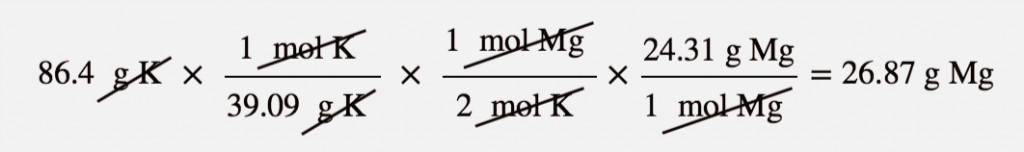Test Yourself

What mass of H2 will be produced when 122 g of Zn are reacted?

Zn(s) + 2 HCl(aq) $\longrightarrow$ ZnCl2(aq) + H2(g)

3.77 g

### Example 10

Interpret this balanced chemical equation in terms of moles.

P4 + 5 O2 $\longrightarrow$ P4O10

Solution

The coefficients represent the number of moles that react, not just molecules. We would speak of this equation as “one mole of molecular phosphorus reacts with five moles of elemental oxygen to make one mole of tetraphosphorus decoxide.”

Test Yourself

Interpret this balanced chemical equation in terms of moles.

N2 + 3 H2 $\longrightarrow$ 2 NH3

One mole of elemental nitrogen reacts with three moles of elemental hydrogen to produce two moles of ammonia.

### Example 11

For the balanced chemical equation

2 C4H10(g) + 13 O2 $\longrightarrow$ 8 CO2(g) + 10 H2O(ℓ)

if 154 mol of O2 are reacted, how many moles of CO2 are produced?

Solution

We are relating an amount of oxygen to an amount of carbon dioxide, so we need the equivalence between these two substances. According to the balanced chemical equation, the equivalence is 13 mol O2 to 8 mol CO2

We can use this equivalence to construct the proper conversion factor. We start with what we are given and apply the conversion factor: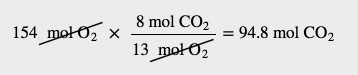The mol O2 unit is in the denominator of the conversion factor so it cancels. Both the 8 and the 13 are exact numbers, so they don’t contribute to the number of significant figures in the final answer.

Test Yourself

Using the above equation, how many moles of H2O are produced when 154 mol of O2 react?

118 mol

## Key Concepts and Summary

A balanced chemical equation may be used to describe a reaction’s stoichiometry (the relationships between amounts of reactants and products). Coefficients from the equation are used to derive stoichiometric factors that subsequently may be used for computations relating reactant and product masses, molar amounts, and other quantitative properties.

### Exercises

1. Determine the number of moles and the mass requested for each of the following reactions: (Hint: Write the balanced equation for each before attempting calculations.)

a) The number of moles and the mass of chlorine, Cl2, required to react with 10.0 g of sodium metal, Na, to produce sodium chloride, NaCl.

b) The number of moles and the mass of oxygen formed by the decomposition of 1.252 g of mercury(II) oxide.

c) The number of moles and the mass of sodium nitrate, NaNO3, required to produce 128 g of oxygen. (NaNO2 is the other product.)

d) The number of moles and the mass of carbon dioxide formed by the combustion of 20.0 kg of carbon in an excess of oxygen.

e) The number of moles and the mass of copper(II) carbonate needed to produce 1.500 kg of copper(II) oxide. (CO2 is the other product.)

f)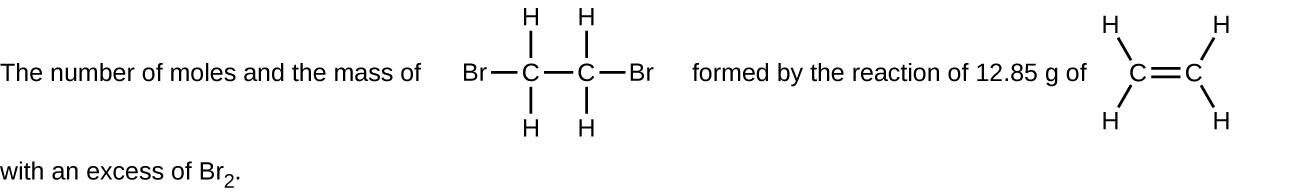2. Determine the number of moles and the mass requested for each of the following reactions: (Hint: Write the balanced equation for each before attempting calculations.)

a) The number of moles and the mass of Mg required to react with 5.00 g of HCl and produce MgCl2 and H2.

b) The number of moles and the mass of oxygen formed by the decomposition of 1.252 g of silver(I) oxide.

c) The number of moles and the mass of magnesium carbonate, MgCO3, required to produce 283 g of carbon dioxide. (MgO is the other product.)

d) The number of moles and the mass of water formed by the combustion of 20.0 kg of acetylene, C2H2, in an excess of oxygen.

e) The number of moles and the mass of barium peroxide, BaO2, needed to produce 2.500 kg of barium oxide, BaO (O2 is the other product.)

f)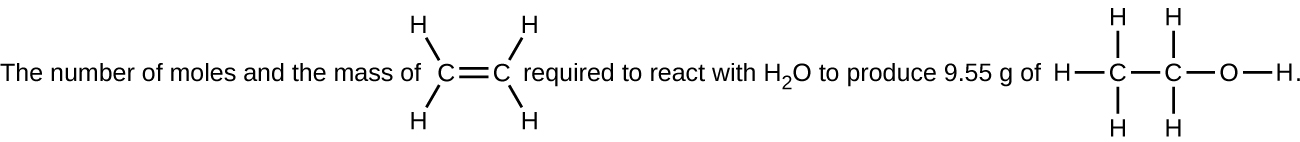3. Gallium chloride is formed by the reaction of 2.6 L of a 1.44 M solution of HCl according to the following equation: $2\text{Ga} + 6\text{HCl} \longrightarrow 2\text{GaCl}_3 + 3\text{H}_2$.

a) Outline the steps necessary to determine the number of moles and mass of gallium chloride.

b) Perform the calculations outlined.

4. Silver is often extracted from ores such as K[Ag(CN)2] and then recovered by the reaction
$2 \text{K} [\text{Ag(CN)}_2](aq) + \text{Zn}(s) \longrightarrow 2\text{Ag}(s) + \text{Zn(CN)}_2(aq) + 2\text{KCN}(aq)$

a) How many molecules of Zn(CN)2 are produced by the reaction of 35.27 g of K[Ag(CN)2]?

b) What mass of Zn(CN)2 is produced?

5. Carborundum is silicon carbide, SiC, a very hard material used as an abrasive on sandpaper and in other applications. It is prepared by the reaction of pure sand, SiO2, with carbon at high temperature. Carbon monoxide, CO, is the other product of this reaction. Write the balanced equation for the reaction, and calculate how much SiO2 is required to produce 3.00 kg of SiC.

6. Urea, CO(NH2)2, is manufactured on a large scale for use in producing urea-formaldehyde plastics and as a fertilizer. What is the maximum mass of urea that can be manufactured from the CO2 produced by combustion of 1.00×103kg1.00×103kg of carbon followed by the reaction?
$\text{CO}_2(g) + 2\text{NH}_3(g) \longrightarrow {\text{CO(NH}_2})_2(s) + \text{H}_2 \text{O}(l)$

7. A compact car gets 37.5 miles per gallon on the highway. If gasoline contains 84.2% carbon by mass and has a density of 0.8205 g/mL, determine the mass of carbon dioxide produced during a 500-mile trip (3.785 liters per gallon).

8. What volume of a 0.2089 M KI solution contains enough KI to react exactly with the Cu(NO3)2 in 43.88 mL of a 0.3842 M solution of Cu(NO3)2?$2 \text{Cu(NO}_3)_2 + 4\text{KI} \longrightarrow 2\text{CuI} + \text{I}_2 + 4{\text{KNO}_3}$

9. The toxic pigment called white lead, Pb3(OH)2(CO3)2, has been replaced in white paints by rutile, TiO2. How much rutile (g) can be prepared from 379 g of an ore that contains 88.3% ilmenite (FeTiO3) by mass?$2\text{FeTiO}_3 + 4\text{HCl} + \text{Cl}_2 \longrightarrow 2\text{FeCl}_3 + 2\text{TiO}_2 + 2\text{H}_2 \text{O}$

10. Think back to the pound cake recipe. What possible conversion factors can you construct relating the components of the recipe?

11. What are all the conversion factors that can be constructed from the balanced chemical reaction 2H2(g) + O2(g) $\longrightarrow$ 2H2O(ℓ)?

12. Given the chemical equation

Na(s) + H2O(ℓ) $\longrightarrow$ NaOH(aq) + H2(g)

a)  Balance the equation.

b)  How many molecules of H2 are produced when 332 atoms of Na react?

13. For the balanced chemical equation

6 H+(aq) + 2 MnO4(aq) + 5 H2O2(ℓ) $\longrightarrow$ 2 Mn2+(aq) + 5 O2(g) + 8 H2O(ℓ)

how many molecules of H2O are produced when 75 molecules of H2O2 react?

14.  Given the balanced chemical equation

Fe2O3(s) + 3SO3(g) $\longrightarrow$ Fe2(SO4)3

how many molecules of Fe2(SO4)3 are produced if 321 atoms of S are reacted?

15.  For the balanced chemical equation

Fe2O3(s) + 3 SO3(g) $\longrightarrow$ Fe2(SO4)3

suppose we need to make 145,000 molecules of Fe2(SO4)3. How many molecules of SO3 do we need?

16.  Construct the three independent conversion factors possible for these two reactions:

2 H2 + O2 $\longrightarrow$ 2 H2O

H2 + O2 $\longrightarrow$ H2O2

Why are the ratios between H2 and O2 different?

The conversion factors are different because the stoichiometries of the balanced chemical reactions are different.

17. What mass of CO2 is produced by the combustion of 1.00 mol of CH4?

CH4(g) + 2 O2(g) $\longrightarrow$ CO2(g) + 2 H2O(ℓ)

18. What mass of HgO is required to produce 0.692 mol of O2?

2 HgO(s) $\longrightarrow$ 2 Hg(ℓ) + O2(g)

19. How many moles of Al can be produced from 10.87 g of Ag?

Al(NO3) 3(s) + 3 Ag $\longrightarrow$ Al + 3 AgNO3

20. How many moles of O2 are needed to prepare 1.00 g of Ca(NO3)2?

Ca(s) + N2(g) + 3 O2(g) $\longrightarrow$ Ca(NO3) 2(s)

21. What mass of O2 can be generated by the decomposition of 100.0 g of NaClO3?

2 NaClO3 $\longrightarrow$ 2 NaCl(s) + 3 O2(g)

22. What mass of Fe2O3 must be reacted to generate 324 g of Al2O3?

Fe2O3(s) + 2 Al(s) $\longrightarrow$ 2 Fe(s) + Al2O3(s)

23. What mass of MnO2 is produced when 445 g of H2O are reacted?

H2O(ℓ) + 2 MnO4(aq) + Br(aq) $\longrightarrow$ BrO3(aq) + 2 MnO2(s) + 2 OH(aq)

24. If 83.9 g of ZnO are formed, what mass of Mn2O3 is formed with it?

Zn(s) + 2 MnO2(s) $\longrightarrow$ ZnO(s) + Mn2O3(s)

25. If 88.4 g of CH2S are reacted, what mass of HF is produced?

CH2S + 6 F2 $\longrightarrow$ CF4 + 2 HF + SF6

26. Express in mole terms what this chemical equation means.

CH4 + 2O2 $\longrightarrow$ CO2 + 2H2O

27. How many molecules of each substance are involved in the equation in Exercise 1 if it is interpreted in terms of moles?

28. For the chemical equation

2 C2H6 + 7 O2 $\longrightarrow$ 4 CO2 + 6 H2O

what equivalences can you write in terms of moles? Use the ⇔ sign.

29. Write the balanced chemical reaction for the combustion of C5H12 (the products are CO2 and H2O) and determine how many moles of H2O are formed when 5.8 mol of O2 are reacted.

30. For the balanced chemical equation

3 Cu(s) + 2 NO3(aq) + 8 H+(aq) $\longrightarrow$ 3 Cu2+(aq) + 4 H2O(ℓ) + 2 NO(g)

how many moles of Cu2+ are formed when 55.7 mol of H+ are reacted?

31. For the balanced chemical reaction

4 NH3(g) + 5 O2(g) $\longrightarrow$ 4 NO(g) + 6 H2O(ℓ)

how many moles of H2O are produced when 0.669 mol of NH3 react?

32. For the balanced chemical reaction

4 KO2(s) + 2 CO2(g) $\longrightarrow$ 2 K2CO3(s) + 3 O2(g)

determine the number of moles of both products formed when 6.88 mol of KO2 react.

1. a) 0.435 mol Na, 0.217 mol Cl2, 15.4 g Cl2

b) 0.005780 mol HgO, 2.890 × 10−3 mol O2, 9.248 × 10−2 g O2

c) 8.00 mol NaNO3, 6.8 × 102 g NaNO3

d) 1665 mol CO2, 73.3 kg CO2

e) 18.86 mol CuO, 2.330 kg CuCO3

f) 0.4580 mol C2H4Br2, 86.05 g C2H4Br2

2. a) 0.0686 mol Mg, 1.67 g Mg

b) 2.701 × 10−3 mol O2, 0.08644 g O2

c) 6.43 mol MgCO3, 542 g MgCO3

d) 713 mol H2O, 12.8 kg H2O

e) 16.31 mol BaO2, 2762 g BaO2

f) 0.207 mol C2H4, 5.81 g C2H4

3. a) $\text{volume HCl solution} \longrightarrow \text{mol HCl} \longrightarrow \text{mol GaCl}_3$; b) 1.25 mol GaCl3, 2.2 × 102 g GaCl3

4. a) 5.337 × 1022 molecules      b) 10.41 g Zn(CN)2

5. $\text{SiO}_2 + 3\text{C} \longrightarrow \text{SiC} + 2\text{CO}$, 4.50 kg SiO2

6. 5.00 × 103 kg

7. 1.28 × 105 g CO2

8. 161.40 mL KI solution

9. 176 g TiO2

10.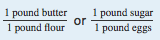are two conversion factors that can be constructed from the pound cake recipe. Other conversion factors are also possible.

11.

12.  2Na(s) + 2H2O(ℓ) $\longrightarrow$ 2NaOH(aq) + H2(g) and 166 molecules

13. 120 molecules
14. 107 molecules
15. 435,000 molecules
16.

17. 44.0 g

18. 3.00 × 102 g

19. 0.0336 mol

20. 0.0183 mol

21. 45.1 g

22. 507 g

23. 4.30 × 103 g

24. 163 g

25. 76.7 g

26. One mole of CH4 reacts with 2 mol of O2 to make 1 mol of CO2 and 2 mol of H2O.

27. 6.022 × 1023 molecules of CH4, 1.2044 × 1024 molecules of O2, 6.022 × 1023 molecules of CO2, and 1.2044 × 1024 molecules of H2O

28. 2 mol of C2H6 to 7 mol of O2 to 4 mol of CO2 to 6 mol of H2O

29. C5H12 + 8 O $\longrightarrow$ 5CO2 + 6H2O; 4.4 mol

30. 20.9 mol

31. 1.00 mol

32. 3.44 mol of K2CO3; 5.16 mol of O2

## Glossary

stoichiometric factor: ratio of coefficients in a balanced chemical equation, used in computations relating amounts of reactants and products

stoichiometry: relationships between the amounts of reactants and products of a chemical reaction